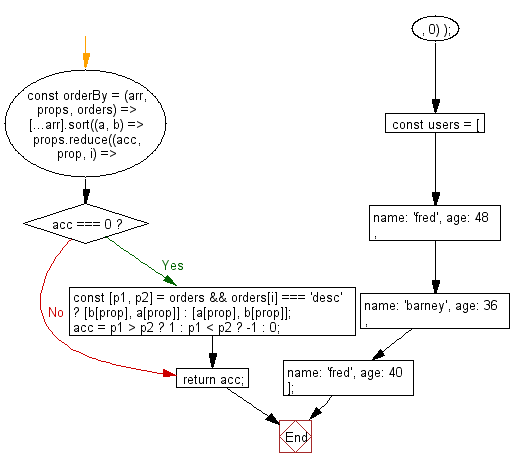# JavaScript fundamental: Get a sorted array of objects ordered by properties and orders

## JavaScript fundamental (ES6 Syntax): Exercise-37 with Solution

Write a JavaScript program to get a sorted array of objects ordered by properties and orders.

• Uses Array.prototype.sort(), Array.prototype.reduce() on the props array with a default value of 0.
• Use array destructuring to swap the properties position depending on the order supplied.
• If no orders array is supplied, sort by 'asc' by default.

Sample Solution:

JavaScript Code:

``````//#Source https://bit.ly/2neWfJ2
const orderBy = (arr, props, orders) =>
[...arr].sort((a, b) =>
props.reduce((acc, prop, i) => {
if (acc === 0) {
const [p1, p2] = orders && orders[i] === 'desc' ? [b[prop], a[prop]] : [a[prop], b[prop]];
acc = p1 > p2 ? 1 : p1 < p2 ? -1 : 0;
}
return acc;
}, 0)
);
const users = [{ name: 'fred', age: 48 }, { name: 'barney', age: 36 }, { name: 'fred', age: 40 }];

console.log(orderBy(users, ['name', 'age'], ['asc', 'desc']));
console.log(orderBy(users, ['name', 'age']));
```
```

Sample Output:

```[{"name":"barney","age":36},{"name":"fred","age":48},{"name":"fred","age":40}]
[{"name":"barney","age":36},{"name":"fred","age":40},{"name":"fred","age":48}]
```

Flowchart:Live Demo:

See the Pen javascript-basic-exercise-1-37 by w3resource (@w3resource) on CodePen.

Improve this sample solution and post your code through Disqus

What is the difficulty level of this exercise?

Test your Programming skills with w3resource's quiz.

﻿

## JavaScript: Tips of the Day

Chunks an array into n smaller arrays

Example:

```const tips_chunkIntoN = (arr, n) => {
const size = Math.ceil(arr.length / n);
return Array.from({ length: n }, (v, i) =>
arr.slice(i * size, i * size + size)
);
}
console.log(tips_chunkIntoN([1, 2, 3, 4, 5, 6, 7,8], 4));
```

Output:

```[[1,2],[3,4],[5,6],[7,8]]
```